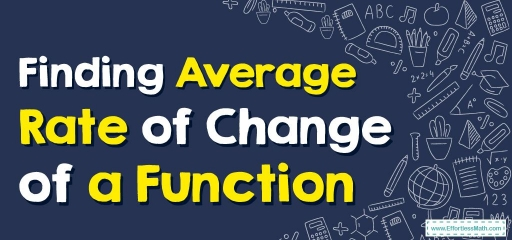# How to Find Average Rate of Change of a Function?

The average rate of change is the rate at which one value within a function changes relative to another. Here you will learn how to find the average rate of change of a function.What is the average rate of change? It is a measure of how much the function changes per unit, on average, over the interval. It is derived from the slope of the straight line that connects the endpoints of the interval in the function diagram.

## A step-by-step guide to the average rate of change of a function

The average rate of change function is defined as the average rate at which one quantity is changing for something else changing. In other words, an average rate of change function is a process that calculates the amount of change in one item divided by the corresponding amount of change in another.

We can define the average rate of change of a function $$f$$ from $$a$$ to $$b$$ as:

$$\color{blue}{ A(x) =\frac{f(b)-f(a)}{b-a}}$$

Where,
$$f(a)$$ and $$f(b)$$ are the values of the function $$f(x)$$ at $$a$$ and $$b$$ respectively.

### Average Rate of Change of a Function – Example 1:

Calculate the average rate of change of a function, $$f(x)=3x+10$$ as $$x$$ changes from $$5$$ to $$8$$.

Solution:

First, find the $$f(5)$$:

$$f(x)=3x+10$$ → $$f(5)=3(5)+10$$ →$$f(5)=25$$

Then, find the $$f(8)$$:

$$f(x)=3x+10$$ → $$f(8)=3(8)+10$$ →$$f(8)=34$$

Now, use the average rate of change formula: $$\color{blue}{ A(x) =\frac{f(b)-f(a)}{b-a}}$$

$$A(x)=\frac{f(8)-f(5)}{8-5}$$

$$=\frac{34-25}{8-5}=\frac{9}{3}$$

$$=3$$

## Exercises for Average Rate of Change of a Function

### Calculate the average rate of change of each function.

1. $$\color{blue}{\:f\left(x\right)=x^2-8x,\:2\le \:x\le 6}$$
2. $$\color{blue}{h\left(x\right)=\left(x+4\right)^2+5,\:−5\le \:x\le −1}$$
3. $$\color{blue}{g\left(x\right)=x^2+3x+1,\:\left[0,\:2\right]}$$
4. $$\color{blue}{\:f\left(x\right)=1-5x-x^3,\:\left[1,\:3\right]}$$
1. $$\color{blue}{0}$$
2. $$\color{blue}{2}$$
3. $$\color{blue}{5}$$
4. $$\color{blue}{-18}$$

### What people say about "How to Find Average Rate of Change of a Function? - Effortless Math: We Help Students Learn to LOVE Mathematics"?

No one replied yet.

X
30% OFF

Limited time only!

Save Over 30%

SAVE $5 It was$16.99 now it is \$11.99